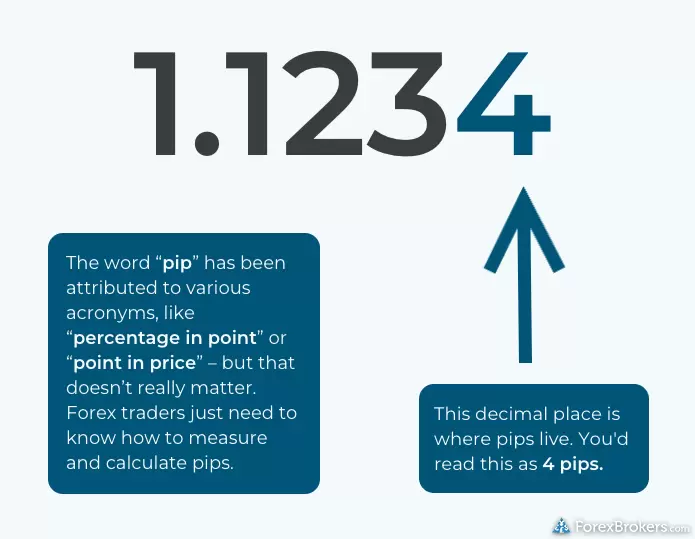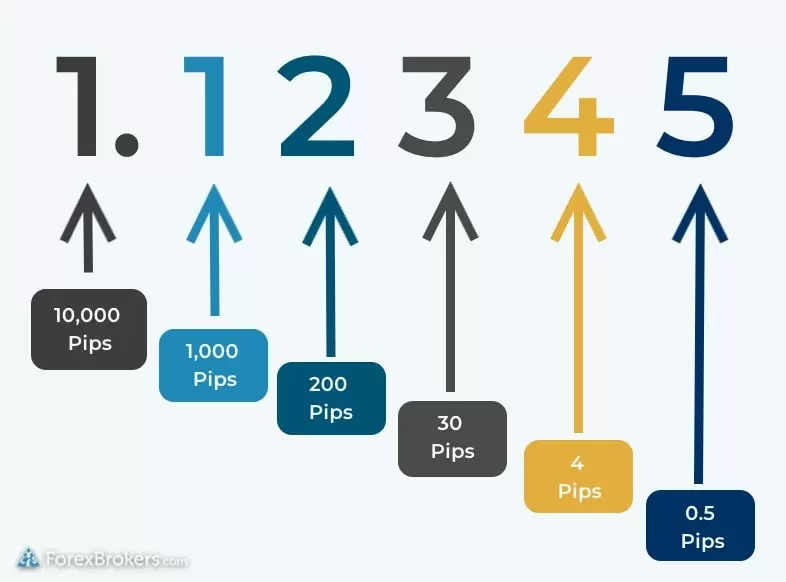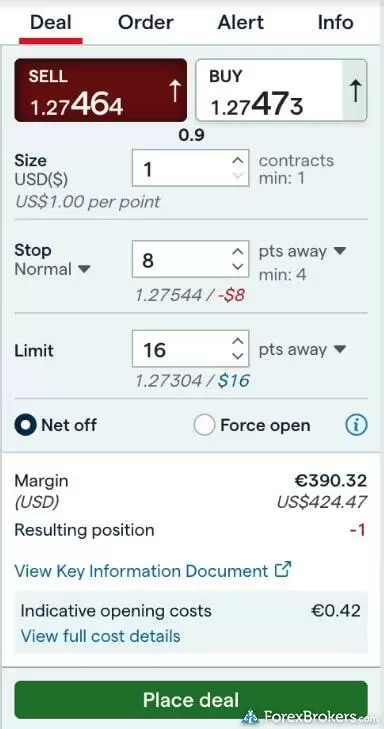ForexBrokers.com is committed to the highest ethical standards and reviews services independently. Learn How We Make Money
Intro to Forex

# What is a Pip?

October 12, 2023

Forex traders need to be able to monitor tiny movements in the exchange rates of international currencies. When I say tiny, I mean movements as small as hundredths (or even thousandths) of a cent. You may have read or heard about pips in the forex market; pips are just a way for forex traders to talk about (and measure) those incredibly small price shifts in the forex market.

It’s essential that forex traders understand what pips are and how they work. Even a single pip can convey tremendous value, depending on the size of a given trade. Let’s dive into the ins and outs of pips in the forex market.

## What is a pip?

A pip is an important unit of measurement used for tracking minuscule changes in the price of a currency. Pips are small enough to measure fractions of a cent; they provide forex market participants and forex traders with an incredible degree of price precision when quoting exchange rates or valuing currencies. One pip is the equivalent of one one-hundredth of a percent (1/100th of 1%).

## How are pips used?

The global forex market operates 24 hours a day and currency exchange rates are in a continual state of flux. Pips enable forex traders to make currency trades based on price movements as small as 1/10,000th of a currency unit (that’s 1/100th of one cent in U.S. dollars). That level of granularity in pricing data creates an almost limitless number of trading opportunities for forex traders; because forex prices are quoted in pips, prices can change hundreds of times per minute.

Typically, a pip represents 1/10,000th of a currency unit. This is the case for currencies that are denominated in pennies or cents, such as the dollar or the euro. In some cases, currencies are already denominated in their smallest unit. The Japanese yen, for example, does not have smaller denominations than “one yen”; these are also known as zero-decimal currencies. For the Japanese yen, a pip would be one 100th of a unit of currency.

If you want to learn how to read pips, it’s helpful to remember that – for most currencies – they are represented in the fourth decimal place of most quoted exchange rates (1/10,000th = 0.0001).## How to read pips within a currency exchange rate

Say you’re using your broker’s forex trading platform and you see that the exchange rate for the EUR/USD is 1.0794. At that exchange rate, you’ll pay \$1.0794 U.S. dollars for each euro. Later in the day, you see that the exchange rate went from 1.0794 to 1.0795.

This is an exchange rate increase of one pip.

If the exchange rate went from 1.0794 to 1.0799, that would be an increase of five pips.

directions_runExchange rates can change quickly

Exchange rates are in a constant state of flux; the price of a currency can change hundreds of times a minute.

Now, let’s imagine the exchange rate includes one more decimal place. You look at the exchange rate for the EUR/USD and see that it is displayed as 1.12345. This extra decimal place is known as a fractional pip. For an exchange rate of 1.12345, the “5” would represent 0.5 pips. The graphic below illustrates how to read an exchange rate in pips:A forex trader reading the above exchange rate for the EUR/USD would see that the price for each euro in U.S. dollars would be \$1.12345.

I mentioned above that this works differently for zero-decimal currencies like the Japanese yen. For a currency pair such as the USD/JPY, the exchange rate would look more like this: 123.45.

Remember: There are fewer decimal places for the USD/JPY pair because the yen is already denominated in its smallest unit. One pip for the Japanese yen is 1/100th of a yen, so if the exchange rate is 123.45, the “5” represents five pips.

## How to calculate the value of a pip

Let’s explore the formula used to calculate pip values.

It’s important to remember that the value of a pip scales with the size of each forex trade. The larger the trade value and trade size, the larger the value of each pip (and vice versa).

When you place an order to buy or sell a forex pair, the value of your trade size will dictate the value of each pip (your margin is also calculated based on the value of your trade size).

For example, if you sell 100,000 EUR/USD at an exchange rate of 1.1234, that trade is worth \$112,340.00 and each pip will be worth roughly \$11.23.

Formula: To determine the value of a pip for a given currency pair, take the pip format for the pair in question (0.0001, in the example above) and multiply it by the current exchange rate (our example exchange rate above was 1.1234). Then, multiply that figure by the trade size. This will give you the value of one pip for that specific trade.

Let’s break that down:

The pip format is 0.0001, and our example exchange rate is 1.1234.

0.0001 x 1.1234 = 0.00011234 pips.

Our trade size will be 100,000 units.

0.00011234 x 100,000 = 11.234.

For this trade, one pip will be worth roughly \$11.23.

A general rule of thumb: As some currency pairs result in a pip value that is less than \$1, many traders will simply approximate that a single pip is roughly equivalent to about \$1 per 10,000 units of currency. This scales linearly as follows:

• Micro trade size of 1,000 units: pip value roughly equivalent to 10 cents.
• Mini trade size of 10,000 units: pip value roughly equivalent to 1 dollar.
• Standard trade size of 100,000 units: pip value roughly equivalent to 10 dollars.

## How do pips relate to the spread?

The spread is a measurement in pips of the difference (or distance) between the bid price and the ask price.

When looking at a currency pair in your broker’s trading platform, you’ll see exchange rates for both the bid price and the ask price. The bid price represents the exchange rate for selling, and the ask price is the exchange rate for buying.

Calculating the spread is really simple. All you need to do is subtract the bid price from the ask price.

Let’s look at a hypothetical example.

Bid price exchange rate for the EUR/USD: 1.0877

Ask price exchange rate for the EUR/USD: 1.0879

Calculating the spread: 1.0879 - 1.0877 = 0.0002 (2 pips).

## What is the best forex pip calculator?

Online pip calculators on can be used for research or educational purposes. Here at ForexBrokers.com, we've developed our own pip calculator. Feel free to give it a try:

ForexBrokers.com Pip Calculator

That said, some of my favorite pip calculators are incorporated into online trading platforms within the trade ticket. For example, the interface on IG’s web platform will show you the pip value in real time as you change the size of your order.## More educational resources for new forex traders

Developing a solid understanding of pips is a huge milestone in your journey to becoming a forex trader. Now that we've covered some of the basics, we'll move on to a more complicated subject: leverage in the forex market. Leverage can be a bit trickier to understand at first, but once you've wrapped your head around using a margin account to trade with leverage you'll be one step closer to getting started as a forex trader.

❮    Previous